Pathways To Learning... Since 2005 Hong Kong Registered School 566985

# B - back-substitution to brackets - Mathematics Dictionary

A   B   C   D   E   F   G   H   I   J   K   L   M   N   O   P   Q   R   S   T   U   V   W   X   Y   Z

back-substitution: A method of solving a system of linear equations (or the equivalent matrix equation) that involves finding the components in the reverse order to the way it is indexed, due to its being manipulated to a row-echelon form. (The Upper-triangular form of a square matrix is a special case of this.)

backward difference: A backward difference considers the absolute or directed difference between the value of a function (thus including the terms of a sequence) with a particular argument and the correponding value at a fixed interval prior to the nominated argument. With the following notations, f(x) is the function in question, x is the argument, h is the fixed interval while the nabla symbol (upside-down Greek capital Delta) represents the backward difference.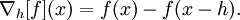ball In mathematics, a ball refers to the collection of all points within a fixed distance of a fixed point. It differs from a sphere in that a ball include all points in the interior (so that the distance between the points and the fixed point - the centre - can be anything less than or equal to a certain value) while a sphere includes only points on the "surface" (so that the distance between the points and the fixed point - the centre - can only be exactly the same as the given value.)

ballistics: The mathematical study of projectiles.

bar: A unit of pressure roughly based on atmospheric pressure at sea level.

bar chart: A graphical representation of data using rectangular "bars" where the height (or length) of the vertical (or horizontal) bars are proportional to the frequency.

barycentre: The centre of mass of an object. The point (not necessarily within the object) which can be used to calculate linear motion of a rigid body as if all of the object's mass are at that point (the centre of mass) only. (Considering the object as a particle.) This is the basis of Euler's First Law.

barycentric: Of or related to the centre of mass of an object.

base: 1. The number which is raised to a given power. (i.e. in a^b b can be called the exponent/index/power while a is the base.)

2. A line (in a 2D figure) or a plane (in a 3D figure) that is considered perpendicular to the height (or with reference to which we define the height) regardless of the orientation of the object.

basis: (plural bases) A basis of a vector space is a minimal spanning set of vectors. That is, a set of vectors which can be used to represent, in linear combinations, all vectors in that particular vector space in exactly one way. The concept of basis is the generalised idea of a coordinate system.

Bayes factor: The Bayesian equivalent (in its application) to the classical frequentist concept of hypothesis testing.

Bayesian inference: A iterative process of statistical inference where observations are used in inferring parameters of a probability distribution.

bearing: A way of representing directions (horizontal directions: perpendicular to up and down) in navigation where a direction is represented by a three-digit number between 000 and 360, measured from the North clockwise. (So that 000 is North, 090 is East, 180 is South and 270 is West.) Note that conventionally, the number is given in 3 digits even if it is less than 100. And while theoretically, there is no reason why the system be limited to integers, it is most often not needed beyond the degree of accuracy given by integer value bearings.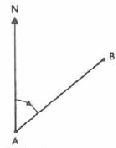becquerel: An SI derived unit of radioacitivity denoted by the symbol Bq.

bell-shaped curve: Informal class="d-title" name given to the normal distribution due to the shape of the graph of the probability density function resembling the shape of a bell. It may be more common to refer to this distribution by this class="d-title" name than as a Normal Distribution in social sciences and non-scientific diciplines.

bend point: Also known as an (extremal) turning point.

Bernoulli distribution: The distribution describing the outcomes of a single Bernoulli trial. (i.e. it is a special case of the binomial distribution when n=1)

Bernoulli's equation: A first-order differential equation whose main application is fluid flow.

Bernoulli trial: A trial with only two possible outcomes, conventionally labelled success or failure unless one needs to be specific within the context, without loss of generality.

Bessel's equation: A second order ordinary differential equation(ODE)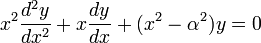whose solutions are the Bessel functions.

beta distribution: A important univariate continuous probability distribution over the open interval (0, 1).

beta function: A symmetric function, denoted by B(x, y), defined by the integral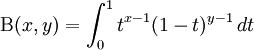The beta function is related to the gamma function by the folowing identity.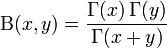bias: 1. A systematic deviation of the inferred expected values from the inferred actual/theoretical value.

2. The factors which caused such deviations.

biconditional: An equivalence relation indicating two mathematical sentences to be necessary and sufficient conditon of each other.

bijection: A one-to-one correspondence of 2 sets. A function that is both an injection and a surjection. That is, a way of identifying the elements from 2 sets with each other so that any element from either set is identified with exactly one element from the other set.

bilateral symmetry: Reflective symmetry. A type of self-similarity through reflection in a line or a plane.

bilinear: The condition of an expression of 2 variables that is linear while considering individually each variable (while the other variable is fixed.) It is possible for an expression to be bilinear while not being linear.

billion: The class="d-title" name variously given to a thousand million and a million million in the past. Traditionally, the US used a billion as the former and the UK as the latter, also referred to as short billion and long billion respectively. With the rapid globalisation in the past few decades, the US definition has become more prevalent although it is better to clarify as its use is dominant while not ubiquitous yet.

bimodal distribution: A distribution with exactly 2 modes. That is, the distribution has exactly 2 values of the highest frequency (for discrete distributions) or frequency density (for continuous distributions).

binary number: A number expressed in base 2, so that each digit represents a power of 2.

binary operation: An operation, such as addition or multiplication, performed on two elements of a set to derive a third element

binary system: A base 2 positional system representing numbers with only the digits 0 and 1.

binary variable: A random variable with only 2 possible outcomes.

binomial: A polynomial with two terms.

binomial coefficients: Numbers used in the calculation of the coefficients of binomial expansions. They can be represented in Pascal's triangle and are identical to the concept of combinations in combinatorics.

The symbol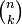is used in the calculation of the (k+1)st term in a binomial expansion of exponent n, it is also the number of ways that a set of k objects can be chosen from a set of n distinct objects, provided that the set of k objects are chosen at the same time and so the order (of being chosen) does not matter.

The calculation of these binomial coefficients involves factorials as follows,Note that the following is true for all values of n>=0binomial distribution: The distribution of a random variable denoting the number of successes in a fixed number of independent Bernoulli trials with constant probability. For n trials and probability p of success in each trial, the probability of k successes is given bybinomial series: The sum of a series that is a binomial expansion. It is mostly of interest when the series is infinite, which means that the exponent in the expansion must not be a non-negative integer.

binomial theorem: A theorem providing the form of the expansion of a binomial expression raised to an arbitrary exponent.This expansion is finite when n is a non-negative integer. (Or in the trivial case when either x or y is zero.)

biquadratic: A polynomial that is the quadratic of a square. The result is a quartic whose cubic and linear coefficients are zero. Note that this term is also used to refer to the product of 2 quadratics or the quadratic of a quadratic, which makes the term equivalent to the term quartic.

birectangular: A plane figure with 2 right angles.

bisect: Dividing into 2 equal halves.

bisector: A line or plane which divides a geometrical object (e.g. a figure or an angle) into 2 congruent parts.

bit: The simplest unit of information consisting of one binary digit, conventionally labelled 0 and 1.

bitangent: The tangent of a curve simultaneously at 2 different points.

bivariate distribution: A distribution of two random variables considered together.

bootstrap: A statistical re-sampling method for obtaining estimators of distribution parameters.

bound: A value or a mathematical object which is related to another mathematical object in a strict (partial) order.

boundary: The exterior of a set. A set which is in the closure (of another set) but not the interior.

boundary conditions: Additional information (constraints) to differential equations regarding the extremes of the domains which help refine a family of solutions.

bounded function: A function with finite bounds.

bounded sequence: A sequence whose set of values are a bounded set.

bounded set: A set is bounded if its closure does not consist of any infinite values.

box-and-whisker diagram: (box plot) A graphical summary of data representing the boundaries of the 4 quadrants on a scale with the middle quadrants represented as rectangles (boxes) and the outer quadrants as lines (whiskers).

box plot: Another class="d-title" name of the box-and-whisker diagram, also known as a box-and-whisker plot.

braces: Symbols { and } commonly used to represent the order of operations along with parentheses and brackets. (And other less common types.) They're also used to represent sets.

brachistochrone: The trajectory of fastest travel between 2 points if a bead is considered to be guided by a smooth wire, under gravity only, between the 2 points starting from rest. Note that the starting point must not be the lower of the 2 points or the bead would never reach the other point. (At the starting point, the bead has zero Kinectic energy. It will never reach the point with higher gravitational potential energy. However, due tot he smoothness of the wire, points of equal heights are possible.)

brackets: Symbols [ and ] commonly used to represent the order of operations, along with parentheses and braces. (And other less common types.) Informally, all 3 pairs of symbols are referred to as brackets. Brackets are also used to represent matrices amongst other mathematical objects.

A   B   C   D   E   F   G   H   I   J   K   L   M   N   O   P   Q   R   S   T   U   V   W   X   Y   Z

Genius is one percent inspiration and ninety-nine percent perspiration.